February 19

# Kalman Filter 101: State Estimation

lang: en_US

Do you know how the state estimation was conceptualized in the Apollo 6 mission in 1960? Let us go back in time and try to understand what complications scientists and researchers faced back then. The trajectory of spacecraft from Earth to the Moon and back had to match the following specifications. The equations of motion used to predict the motion of spacecraft are complex in reality. The spacecraft’s instruments are subjected to random noise and updating measurements occur at irregular intervals. The milestone marked by the Apollo mission enabled humanity’s presence on the Moon, not just in space! This was made possible when NASA’s engineer Stanley F. Schmidt working on this problem came across his acquaintance Rudolph Kalman.Yes, the infamous Rudolph Kalman after whom the Kalman Filter was named. Before we get into the math and deeper understanding of the Kalman Filter, we will get acquainted with a few associated terminologies.

## How do we define the State of a System?

A simple Wikipedia search will tell you “state of a system describes enough about the system to determine its future behavior in the absence of any external forces affecting the system”.

Consider you plan a trip to a wildlife sanctuary. As soon as you are settled in your car and buckled up, you first punch in the coordinates to the location. The GPS pulls out appropriate routes based on traffic and travel time.Your current position is to be updated at say every 10 seconds, to provide a precise route. The knowledge of input acceleration is also essential. The systems whose output depends on input and initial conditions are referred to as systems with memory or dynamic systems. Thus we can say that state contains the past history of the system necessary to determine the system’s response to any given input. Therefore “the complete state of the system can be considered to be a vector having components which are the variables of the system which are closely associated with initial conditions. So a state can be defined as vector X(t) called state vector”, as given in Bakshi. U. A, Bakshi. V. U. Control Systems.

## What is a Kalman Filter?

Now going back to the example we discussed, did you wonder how easy it was to find your way through a deep tunnel?

The sensors present on board a car are IMU (Inertial Measurement Unit), GPS, and Odometer. IMU provides the car’s acceleration and angular velocity using an accelerometer and gyroscope, respectively. The GPS receiver locates the true position of a car on Earth via the signals received from satellites. And relative distance traveled by car is provided by the Odometer. These values are interpreted by the navigation system to estimate (predict) the trajectory.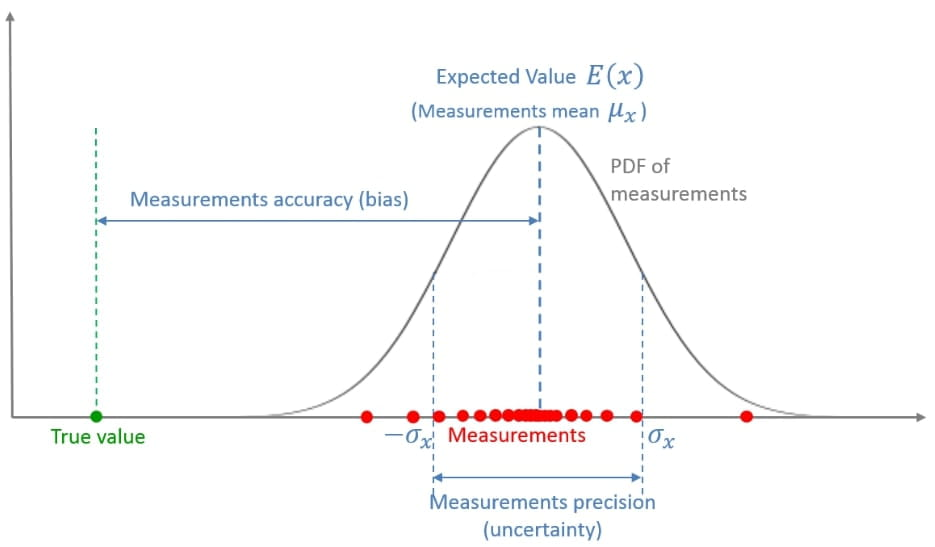Measurement Uncertainty

Generally, natural phenomena follow Normal Distribution, also known as Gaussian Distribution. The Gaussian curve is called Probability Density Function (PDF) for normal distribution. The measurement errors are said to be distributed normally. This assumption is also applicable in the Kalman Filter design.PDF vs Vehicle Position

Consider the previous figure showing the plot of vehicle position against the probability density function (PDF), mean values are given by the center of the bell curves and the spread gives the variance of the associated variables. If we are at position Xt-1 (given in light blue) and we predict the position of the vehicle at Xt (given in blue) using equations of motion. And the measured value is Yt (given in red). The predicted and estimated states have Gaussian shapes as the noise associated with them has a normal distribution. The optimal estimation of the position derived by the Kalman Filter is the weighted average of these two values (given in purple).

The values from IMU and Odometer can be affected by the road conditions and changes in acceleration of the car. GPS data is accurate but the signal might be weak when you are traveling in a tunnel. These account for the Process Noise.

We should also include the Measurement Noise as there is always an uncertainty in the measured variables that arise from the sensors. The data obtained is updated in delayed intervals and has drift errors. The Odometer (or Wheel Speed Sensor) readings will differ from the speed vector of the wheel hub due to the inherent nature of friction between tires and track.

Therefore, to get the optimum solution for predicting the trajectory of our car, we need to combine data from all these sensors. This is made possible by implementing Kalman Filter.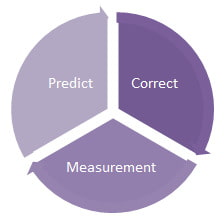Predict-Correct Loop of Kalman Filter

Kalman Filter provides an optimal estimation of a system based on the sensor’s past data and predicts the future position, this process of measuring-correcting-predicting is recursive in nature. Thus, a Kalman Filter is an optimal estimation algorithm, used when the state of the system is measured indirectly. It is commonly applied when measurements from various sensors are available but are subject to noise.

## Math in Kalman Filter

This topic might be daunting for a few, so let’s break down the math part into a few sets of simple equations.

The car dynamic equations are given as: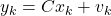, Where

wk

•Process Noise:

•Follows Gaussian distribution

•Mean = 0

•Covariance = R

vk

•Measurement Noise:

•Follows Gaussian distribution

•Mean = 0

•Covariance = Q

And we also have to consider the car model equations: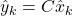Since we have established the presence of measurement noise from sensors and the system’s process noise, we do not know the true position of the car. The Kalman Filter combines measurement and prediction to find the optimal estimate of the car’s true position.

Computationally combining them relates to the multiplication of their PDF which provides the discrete Kalman Filter equation: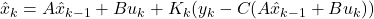This is similar to the state observer equation given by: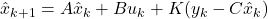Which can be further reduced to obtain the Priori and Posteriori state estimates. A Priori estimate, as the name suggests is calculated prior to/before the current measurement is taken. It predicts the current state by using state estimates from past time step and current input.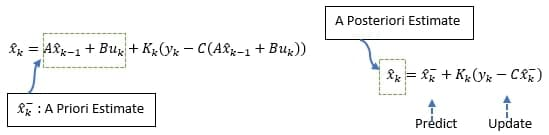Priori Posteriori State Estimates

The A Posteriori estimate uses measurements from sensors and incorporates them into the prediction stage to update the A Priori estimate.

Thus, we can conclude that Kalman Filter is a state observer designed for stochastic systems.

Kalman Filter follows two steps:

• Prediction Step: predicts the future state of the system for given past measurements
• Update Step: estimates the current state of the system for the given measurement at that time step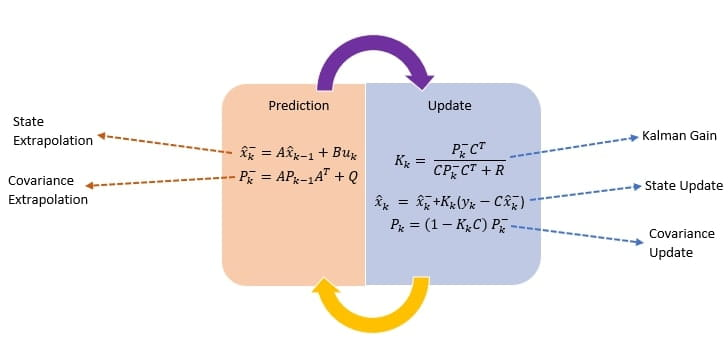Predict-Update Table of Kalman Filter

### Algorithm

• Prediction:
• A Priori state estimate and error covariance P are calculated using the system model
• P is the measure of uncertainty in the estimated state, resulting from process noise
• Past values (k-1) for x hat and p are derived from initial estimates
• Updating:
• A Priori estimate evaluated in the Prediction step is used to calculate x hat and p of A Posteriori estimate
• Kalman Gain is evaluated such that A Posteriori error covariance is low. The correction term ‘C’ in Kalman Gain is weighed to determine if measurement or a priori estimate contributes prominently to the evaluation of x hat.

Do not skip the graphical representation of the working of the Kalman Filter following soon after. Honestly, that took a lot of effort!

### Application of Kalman Filter

The computationally efficient, limited memory requirement Kalman Filter is majorly used in control, navigation, and computer vision applications. From our study thus far, we can deduce that Kalman Filter is used to designing systems with zero bias and minimum variance. Such as:

• Object Tracking to predict the future position of an object, and reduce noise arising from inaccurate detections
• Motion-Based Object(s) Tracking to detect moving objects in each frame, used in computer vision applications like traffic monitoring, face recognition
• Multiple Object Tracking to predict positions associated with possible detections across frames
• Navigation system to derive vehicle’s state, true position, and velocity
• Computer Vision for the feature or cluster tracking is helpful in the identification of human or non-human elements.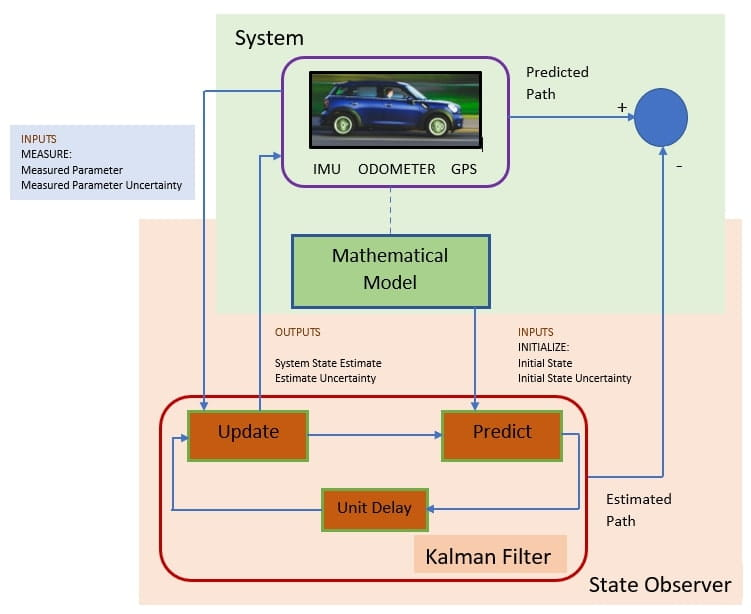Graphical Representation of Implementation of Kalman Filter

## Kalman Filter in MATLAB Simulink

If you have struck this far, then am sure you have been waiting to see the working of the Kalman Filter in MATLAB. So, without further ado let’s jump straight into it!

Melda Ulusoy a renowned staff at Mathworks has created Kalman Filter Virtual Lab. We are going to study the same and understand and design state estimation for a simple linear system comprising a pendulum. The output under consideration is the swing motion of the pendulum modeled using the Simscape Multibody.

Let us first understand the system using the Free Body Diagram and arrive at its state space equations.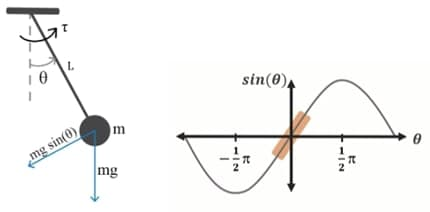Pendulum Oscillation

We are assuming a zero-friction pendulum system. The attribute of interest is the angular position theta. Say that we are using a rotary potential angle sensor to measure this data and that this measurement is noisy. This corresponds to Measurement Noise.

The pendulum is suspended from a rigid body and initiated at an angle, oscillates back and forth due to gravity, before settling down at the center of equilibrium. The otherwise linear system has a non-linearity term given by Sin(theta). For smaller values of theta, the system settles down fast and the function acts linearly. The linearized equation of motion is given below.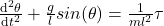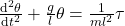We obtain the following equations by replacing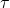with ‘u’ and taking the first state as angular position and the second state as angular velocity.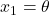;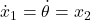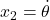;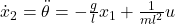The state space matrices are further derived as: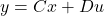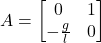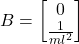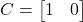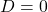Let the fun part begin! We are going to implement this system in Simulink. A pendulum model is created using Simscape Multibody, using which we can define a mechanical system using components such as joints, sensors, bodies, and force elements. The system also solves the equations of motion for the modeled system. We can define the mass, dimensions, and inertia of this pre-built pendulum and the output is the pendulum joint position ‘theta’.

As the first step, we are going to set the angular position parameter to theta0 and angular velocity to theta_dot0 and specify the attributes of the pendulum such as mass, gravity, and length of the pendulum as follows.

% Specify values of parameters for modeling the pendulum

m = 1;       % Pendulum mass [kg]

l = 0.5;     % Pendulum length [m]

g = 9.81;    % Gravity [m/s^2]

theta0 =     pi/18

theta_dot0 = 0

Introduce input torque ‘T’ with zero and also the process noise with the same value. The measurement noise in the system arising from the sensor measurement is provided by Band-Limited White Noise, which is set to 0.000001(1e-6) and sample time to 0.01(1e-2). Combine the measured and actual position values using a Mux and multiply it by a gain of 180/pi to convert the radians reading into degrees. The Demux block is added next to extract individual signals. These individual signals can be studied by connecting them to a Scope separately.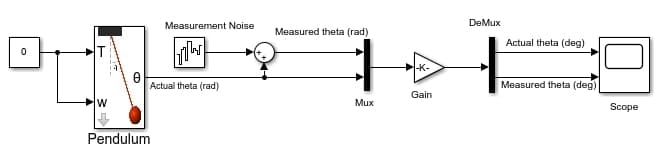Pendulum with Measurement Noise

The noisy angular position output is given in yellow and the actual theta is given in blue color in the following graph.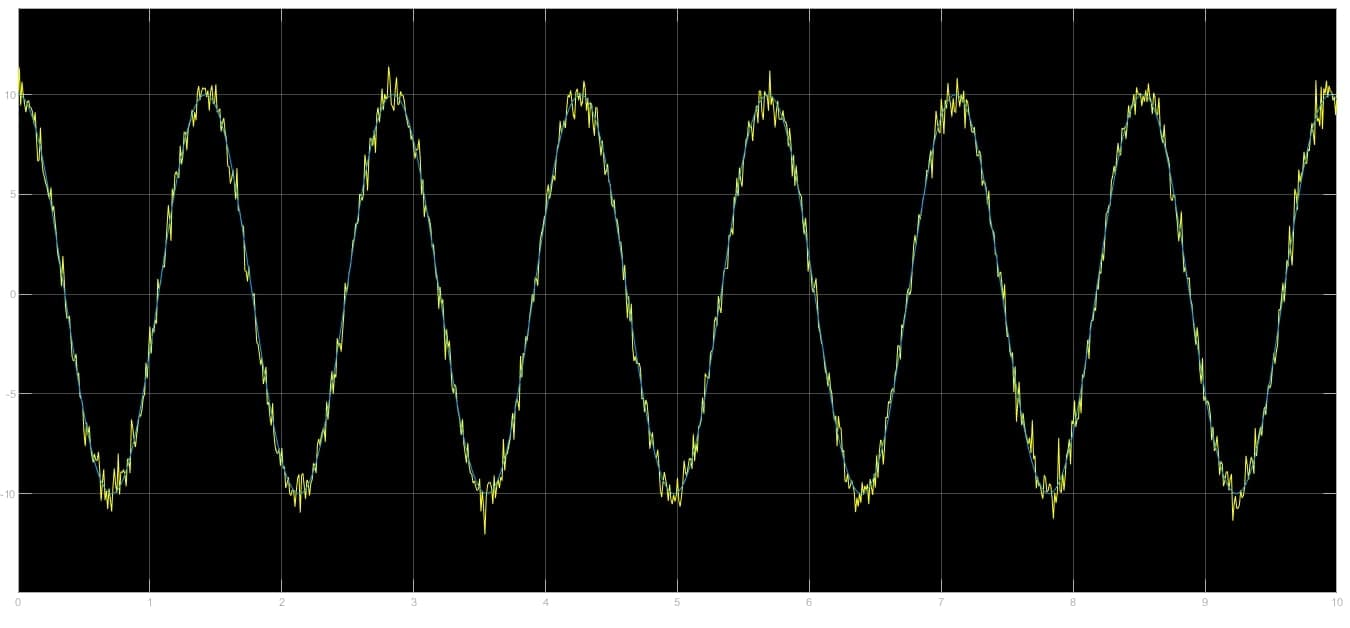Pendulum Output with Measurement Noise

Now let us look at the difference in theta output upon introduction of a Kalman Filter block. It uses the linear model of the system and measurements to compute the optimal state estimates. The block parameter for Kalman Filter is configured as follows:

• Select the Continuous-time domain for the Time domain, when you are not using it in connection with a microcontroller constituting a Discrete system
• Under Model Parameters, opt for Individual A, B, C, D matrices and fill in the appropriate values calculated using mass, length, and gravity defined earlier
• Set the initial state to pi/18 under Initial Estimates
• For Noise Characteristics Q, set the value to diag([0, 1e-3]) for Process Noise Covariance, and the Measurement Noise Covariance represented by R, provide a value 1e-4
• ‘N’ is the cross-covariance matrix, it is used to store the correlation between process and measurement noise. This value is 0 as they are uncorrelated in our example
• Also, change the Process Noise value in the Band-Limited White Noise block to 1e-5 and sample time at 1e-2
• The process noise introduced is assumed to be from a gust of wind

The simulation provides the following feedback:

• Actual theta is given in the dark blue color
• Measurement theta in light blue
• Estimated theta in pinkPendulum connected to Kalman Filter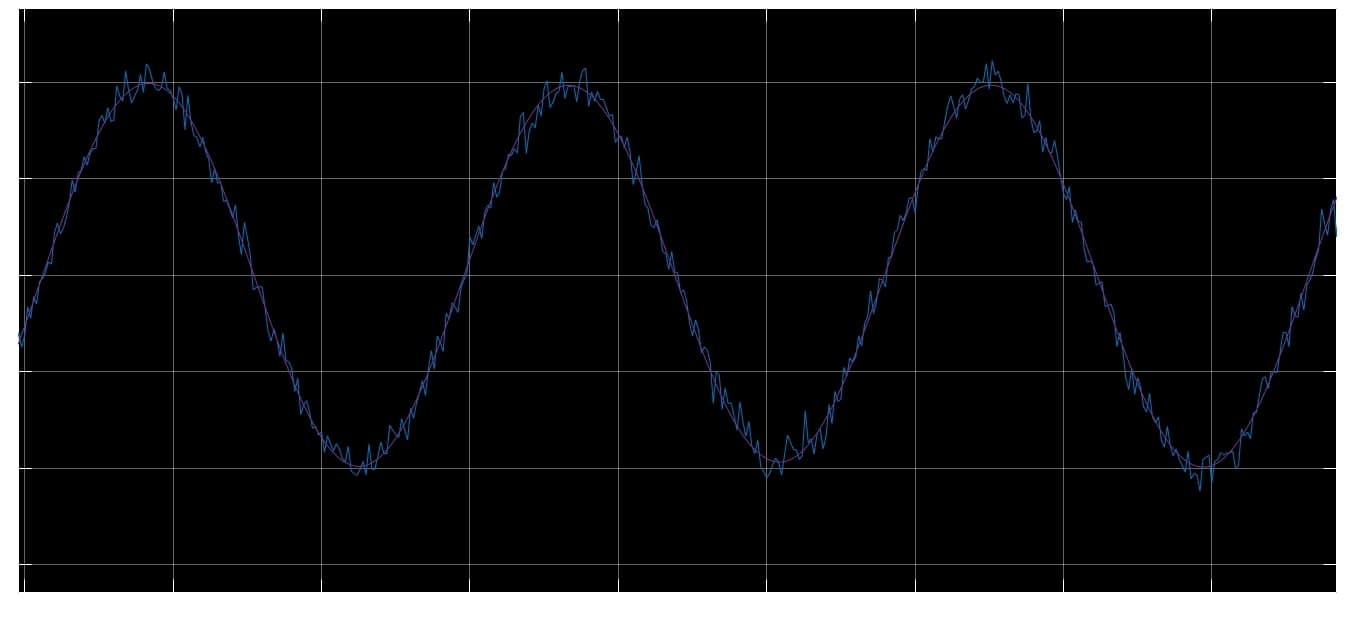Angular Theta Output of Pendulum connected to Kalman Filter

The Kalman Filter design shows good results for small initial values. The system acts non-linearly for large values of theta, and Kalman Filter is specified only for the linear systems. To understand this better, practice this as an exercise and analyze your results: For the above model of a pendulum with Kalman Filter, vary the Measurement and Process Noise covariance and simulate the model. Log your data and compare the angular position output theta. What were your findings?

Did you find a large deviation in your plots at 90 degrees?

So, what can we do about it?

## Extended Kalman Filter to the Rescue!

Once a renowned American Physicist quoted “Of course the word chaos is used in rather a vague sense by a lot of writers, but in physics it means a particular phenomenon, namely that in a non-linear system the outcome is often indefinitely, arbitrarily sensitive to tiny changes in the initial condition”

Every time we are hit with uncertainty, we are up against a nonlinear system. It is the role of an Engineer to think ahead and plan a rescue mission when dealing with a nonlinear system.

Going back to the car example, if we consider the road friction and gust of wind blowing at high speed then, nonlinearity is introduced in our system. Either the state transition function or measurement function or both may be non-linear, which affects state estimation. The solution is to use a non-linear state estimator. An Extended Kalman Filter (EKF) linearizes the non-linear function around the mean of the current state estimates.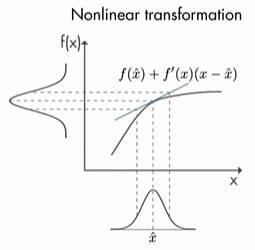Linearization of Non-linear function using Extended Kalman Filter

Linearization is performed at every time step locally resulting in Jacobian matrices which will be used further in predicting-update states of the Kalman Filter.

### Implementation of Extended Kalman Filter in MATLAB

Similar to the Kalman Filter block, MathWorks has designed the Extended Kalman Filter block in its Control System Toolbox. The input to this is the same torque and measured theta, as provided to Kalman Filter. The Extended Kalman Filter block calculates estimates of a discrete non-linear system, so we add a Zero-Order Hold block before the inputs are given directly to the Extended Kalman Filter block.

We are going to specify the state transition function and measurement function as separate .m files in the editor and provide the same file names in the Extended Kalman Filter block under State Transition: Function and Measurement 1: Function, respectively.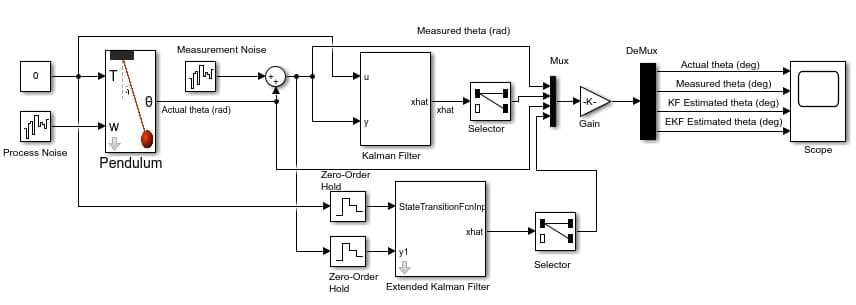Pendulum connected to Extended Kalman Filter

Specify the initial time to [pi/2,0] as we are testing the non-linear system and the Initial Covariance of 1e-6. The Process Noise Covariance, Measurement Noise Covariance, and Sample Time remain same.

With the help of a Mux of 4 input, connect this to the gain block and also increase the input to Scope as 4 and run the simulation.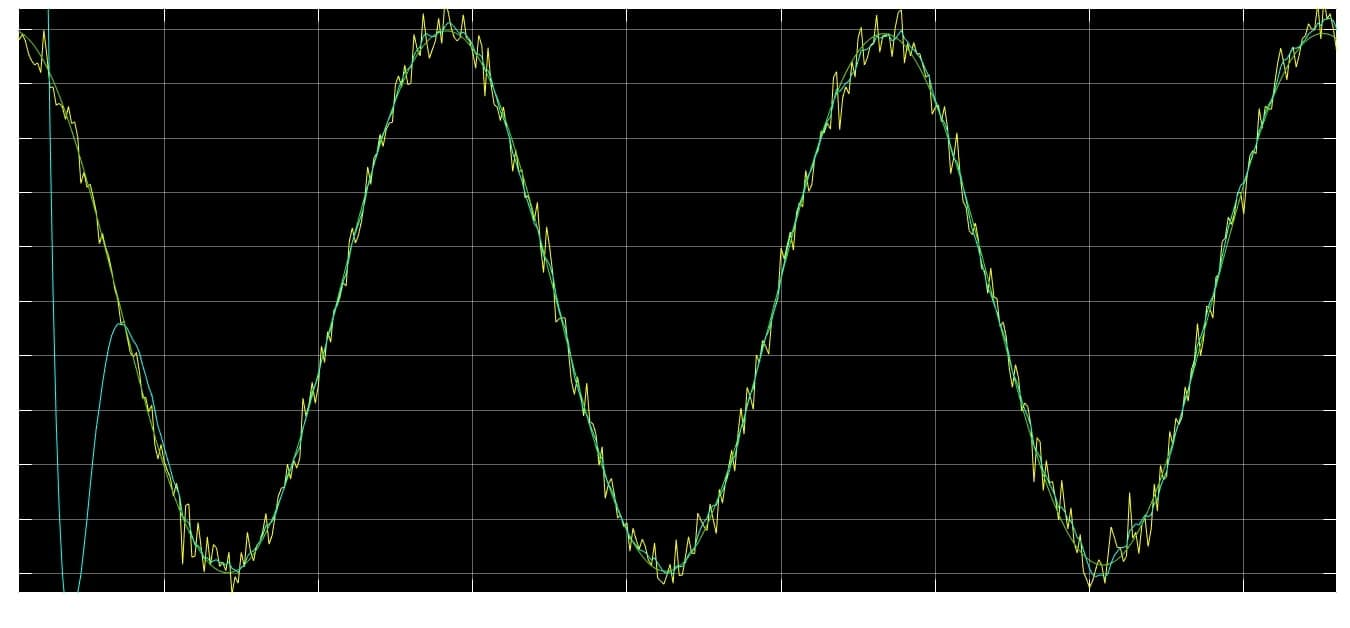Non-linear system Output with Extended Kalman Filter

From the simulation results, we can clearly infer that the pendulum system is no longer non-linear and settles at under 1 sec.

• Yellow is measured theta
• Green is actual theta
• Cyan is estimated theta

### Limitations of Extended Kalman Filter

• It is not an optimal estimator such as Linear Kalman Filter as measurement and state transition model are both non-linear
• For higher-order systems, analytically calculating Jacobians is not easy as complicated derivatives are involved
• In discontinuous systems, Jacobians do not exist as the system is not differentiable
• They are computationally costly
• They are inconsistent statistically as the estimated covariance matrix tends to underestimate the true covariance matrix

## What are Unscented Kalman Filter and Particle Filter?

To overcome the error in state estimation that arises due to non-linearity in either measurement or state transition function or both, estimators and filters were designed that could act on linearized functions of non-linear systems or a system with completely arbitrary values.

Unscented Kalman Filter

Particle Filter

PDF is approxiamted by sampling of points which are Gaussian in nature

Similar to UKF, but  the sampling points approximated can be arbitrary

Numeric approximation of square root of covariance matirx causes stabitility issues

The inexplicit arbitrary nature of distribution requires a large number of particels that may not be easy to handle in every case

## Conclusion

This Blog was written primarily as the first reference for state estimator algorithms present for both linear and non-linear systems.

I am hoping this was extensive enough to get you started with state estimation problems, understand how non-linearity is introduced in a system, and its effects, what filter to use when, and how MATLAB & Simulink has made it easier to model and implement them in both real-time and virtually, for continuous and discrete time domains.

Ready to take your MATLAB skills to the next level? Look no further! At MATLAB Helper, we've got you covered. From free community support to expert help and training, we've got all the resources you need to become a pro in no time. If you have any questions or queries, don't hesitate to reach out to us. Simply post a comment below or send us an email at [email protected].

And don't forget to connect with us on LinkedIn, Facebook, and Subscribe to our YouTube Channel! We're always sharing helpful tips and updates, so you can stay up-to-date on everything related to MATLAB. Plus, if you spot any bugs or errors on our website, just let us know and we'll make sure to fix it ASAP.

Ready to get started? Book your expert help with Research Assistance plan today and get personalized assistance tailored to your needs. Or, if you're looking for more comprehensive training, join one of our training modules and get hands-on experience with the latest techniques and technologies. The choice is yours – start learning and growing with MATLAB Helper today!

Education is our future. MATLAB is our feature. Happy MATLABing!

0 shares

Tags

Extended Kalman Filter, kalman filter, Object tracking, Sensor data, State estimation

#### SANJANA RAMESH TIWARI

MATLAB Developer at MATLAB Helper,
MTech in Sensors and Control Systems,
Cheers to an attempt at combining my love for writing and technology!

## Runge-Kutta method in MATLAB

Runge-Kutta method in MATLAB

## Lidar Point Cloud Data Processing

Lidar Point Cloud Data Processing

#### MATLAB Helper ®

Use Website Chat or WhatsApp at +91-8104622179